## Horizon Culling 2 – The Math

In my previous post on horizon culling we talked about what horizon culling is, the reasons one might want to do it, and went over a simple C# vector class. In this post we’ll take a look at the math we’ll need to use.

In my previous post on horizon culling we talked about what horizon culling is, the reasons one might want to use it, and went over a simple C# vector class. In this post we’ll take a look at the math we’ll need to use.

Referring back to one of our examples from the previous post, it seems like we’d need to calculate the two points where the planet starts curving away – i.e. where the dark blue starts.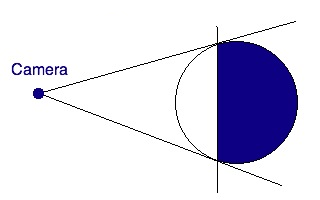That’s the initial approach that I took while figuring this out. Â But it turns out there is a much simpler solution that uses some basic information that we have available to us.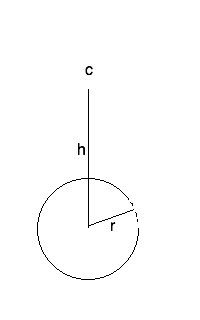In this image, c is the camera position, h is the height of the camera above the center of the planet, and r is the planet radius. These are all things we have readily available. Now let’s make a couple of adjustments…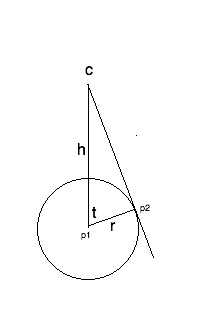If we draw a line segment from the camera to a point tangent to the planet surface, then draw a line from the center to that point, we get a nice pretty right triangle. That makes the angle t look very interesting. That angle represents the point on the horizon where it starts to curve away from what the camera can see. When drawing the planet we can calculate a similar angle for each triangle we’re drawing. Â If that angle is less than t then the triangle is visible to the camera. If it’s greater than t then it’s over the horizon. So, let’s figure out how to calculate that angle.

Dredging through some ancient high school memories we meet up with our old friend Sohcahtoa. I don’t recall the entire story I was taught about Sohcahtoa, but it had something to do with a Native-American (we called them Indians back then) who was gifted at math. It seems there are quite a few different versions of the story, but all you really need to remember is the mnemonic.

We can divide the mnemonic into three sections: soh-cah-toa. The first letter in each section represents a trigonomic function: sin, cosine, and tangent. The next two letters represent the ratio of the two sides of a right triangle that gives you the sin, cosine, and tangent. The letter o stands for the Opposite side, which means the side opposite the angle we’re considering; a is Adjacent, meaning the side adjacent to the angle; h is Hypotenuse, or the longest side. Sohcahtoa expands out to:

Sin = opposite / hypotenuse

Cosine = adjacent / hypotenuse

Tangent = opposite / adjacent

For our planet, the side opposite the angle t is the line segment that runs from C to p2. We don’t know the length of that, so it’s pretty much worthless to us. However, we do know the length of the hypotenuse – it’s the height of the camera from the center. We also know the length of the adjacent side – it’s the planet radius. The only one of our trig functions that utilizes the adjacent side and the hypotenuse is Cosine. The cosine of the angle t is the length of the adjacent side divided by the length of the hypotenuse, or cos(t) = r / h.

What we really want though is the actual angle t, not the cosine. For that we have the handy function called arccosine, which returns the angle given a cosine. So our final formula becomes t = arccos(r / h). In C# this becomes:

float t = Math.Acos(r / h);

And since my brain works in degrees instead of radians, we’ll do that conversion as well:

float t = Math.Acos(r / h) * (180.0f / Math.PI);

One thing that’s interesting is that we don’t ever have to find the point p2. The relationship between radius and height only works for right triangles, and the radius never changes, so if we do the divide we’re always going to get the cosine as if we were working with a right triangle. You’ll be able to visualize this a bit better with the final app since you can see p2 moving as you change the height.

And that’s pretty much it. Next time I’ll post the app that lets us visualize this process and I’ll go over how we can use this angle to skip drawing parts of our planet. For now, here’s a screenshot of the app: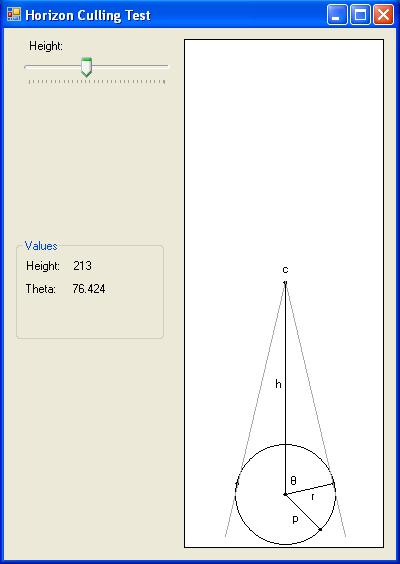Not terribly pretty, but it does the job.

## Horizon Culling

In 3D graphics, a technique that’s often used to improve performance is called culling. Culling means to reduce the population of a wild animal by selective slaughter. It also means, and this is the definition we’ll be using here, to select from a large quantity. For Horizon Culling, the large quantity we’ll be selecting from is the tens of thousands of triangles used to draw a spherical planet. And we’ll be using the horizon to determine which triangles to cull.

This past year I’ve been working on a fractal planet generator using C# and XNA. The system allows transitioning from space all the way down to ground level, and features accurately sized planets, atmospheric scattering shaders, oceans, water reflections, and other typical 3D features. There’s a lot of work to be done to take it where I want to go with it someday, and parts of it don’t work well at all, but it is a good proof of concept and I made huge leaps in understanding 3D graphics techniques. I don’t currently have plans to go over the entire system here, but I’ll pick out parts of it to discuss. And for this post I’ll start with horizon culling.

In 3D graphics, a technique that’s often used to improve performance is called culling. Culling means to reduce the population of a wild animal by selective slaughter. It also means, and this is the definition we’ll be using here, to select from a large quantity. For Horizon Culling, the large quantity we’ll be selecting from is the tens of thousands of triangles used to draw a spherical planet. And we’ll be using the horizon to determine which triangles to cull.

The image below shows why horizon culling is so useful. The camera is looking at the planet, and it can’t see what’s behind the horizon due to the curvature. The dark blue section shows the part of the planet that is invisible to the camera. Since the camera can’t see it, it makes no sense to draw it.The previous image shows that we can eliminate drawing more than half the planet. Now check out the following image. Note that the camera is much closer to the surface of the planet, which means that it can’t see nearly as far before the horizon interferes. This means we can eliminate drawing a huge portion of the planet.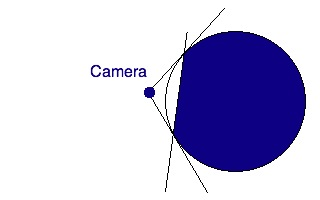The closer you get to the surface of the planet, the less you have to draw. You can use that increased efficiency to draw more detail close to the camera where it matters.

When I was coming up with the algorithm to implement this in my planet generator, I developed a test Windows application that would let me visualize everything in the simplest way I could come up with. Over the next two or three posts I want to do a kind of tutorial and walk you through creating that app. When we’re all through you should have a pretty good idea how the horizon culling works and how to apply the calculations to your app if you’re geeky enough to be creating something to display full sized planets.

Most of the cool things in 3D graphics require math, and this is no exception, but let’s save the math for later. In the remainder of this post we’ll go through the preliminary step of creating a C# Vector class. Now, there’s already a Vector class in XNA that does everything we need, but I wanted the app to work without requiring XNA installation. You may want to read up on vectors if you’re not familiar.

Here’s the Vector class we’ll be using. It’s a basic implementation of only the things that we require in our app and leaves out some useful things such as a cross product. It should be fairly painless to add that sort of thing if you need it.

```public struct Vector
{
public double X; // in most cases you'd probably want to use single precision
public double Y; // floats here, but in my case I needed to be able to position
public double Z; // things at a solar system scale and single just doesn't cut it

public Vector(double X, double Y, double Z)
{
this.X = X;
this.Y = Y;
this.Z = Z;
}

public void SetValue(double X, double Y, double Z)
{
this.X = X;
this.Y = Y;
this.Z = Z;
}

public double Length()
{
return Math.Sqrt(X * X + Y * Y + Z * Z); // length of a 3D vector is sqrt(X^2 + Y^2 + Z^2)
}

public void Normalize()
{
double L = Math.Sqrt(X * X + Y * Y + Z * Z); // get the vector length
X /= L; // divide each component of the vector by the length
Y /= L; // so we end up with a vector of length 1
Z /= L;
}

public static double Dot(ref Vector V1, ref Vector V2)
{
return V1.X * V2.X + V1.Y * V2.Y + V1.Z * V2.Z;
}

public override string ToString()
{
return "&lt;" + X.ToString() + "," + Y.ToString() + "," + Z.ToString() + "&gt;";
}
}
```

You may wonder why this is a struct instead of a class. A struct in C# looks and acts enough like a class that I feel free to call it a class, even though I suppose technically it isn’t. I’ve not really studied into it that much. One big difference though is that structs don’t cause garbage collection because they’re allocated on the stack. Garbage collection is bad in 3D graphics applications. Another way to look at this is that structs act like much like a value (e.g. an int) rather than a reference (e.g. a class, which is in effect a pointer).

I think it’s time to bring this post to a close. In my next couple of posts I’ll go over the details of the culling algorithm and the rest of the app. When we’re all done I’ll post the entire app.

## In the beginning…

I’ve always considered programming an art form. As a result, I try to create code that is clean, readable, and easy to follow – just the way I like my art. I don’t like crappy art, and don’t like crappy code either.

I’ve resisted doing one of these blog things for quite some time now. But lately I’ve felt some motivation to start writing about writing code, and maybe share some of my coding experience.

I got my first computer when I was 12. An Atari 600XL, way back in 1980. I taught myself BASIC, and then 6502 Assembler. I’ve written code in one form or another nearly every day of the 29 years since since then, and enjoyed nearly every minute of it.

I’ve always considered programming an art form. As a result, I try to create code that is clean, readable, and easy to follow – just the way I like my art.  I don’t like crappy art, and don’t like crappy code either.

Of course, now that I’m an old man, I’ve learned that sometimes you have to be practical and just get things done. My code is generally a mix of what I consider some nicely designed parts, and some ugly but functional parts. Although, what comprises good art is pretty subjective, so you as readers may think it’s all ugly crap. Either way, hopefully I can provide some useful information to anyone who is interested.

I’m not 100 percent sure where I want this blog to go. I plan to mostly talk about coding. My whims take me in quite a few directions – sometimes I work on 3D game type things, sometimes websites, sometimes iPhone apps, and any number of other subjects. I’ll probably talk about all of it, and maybe even some non-programming subjects. Generally subjects that are geeky in nature.

Finally, as this thing gets going, bear with the disorganization for awhile, and hopefully at some point geeks of all persuasions will find Crappy Coding an interesting place to spend some time.